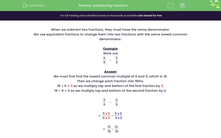# Subtract Fractions

In this worksheet, students will subtract two simple fractions by first finding their lowest common denominator.Key stage:  KS 3

Curriculum topic:   Number

Curriculum subtopic:   Use Four Operations for All Numbers

Difficulty level:#### Worksheet Overview

When we subtract two fractions, they must have the same denominator.

We use equivalent fractions to change them into two fractions with the same lowest common denominator.

Example

Work out

 5 - 5 6 9

We must first find the lowest common multiple of 6 and 9, which is 18.

Then we change each fraction into 18ths.

18 ÷ 6 = 3 so we multiply the top and bottom of the first fraction by 3.

18 ÷ 9 = 2 so we multiply the top and bottom of the second fraction by 2.

 5 - 5 6 9

 = 5 x 3 - 5 x 2 6 x 3 9 x 2

 = 15 - 10 18 18

 = 5 18

Let's have a go at some questions now.

### What is EdPlace?

We're your National Curriculum aligned online education content provider helping each child succeed in English, maths and science from year 1 to GCSE. With an EdPlace account you’ll be able to track and measure progress, helping each child achieve their best. We build confidence and attainment by personalising each child’s learning at a level that suits them.

Get started